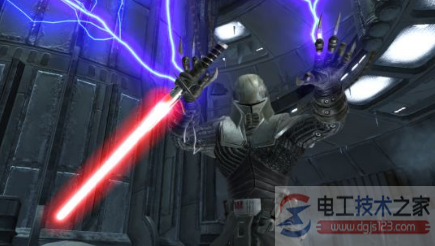# 电流与功率的换算公式_有关电流功率的换算方法

###### 来源：电工天下时间：2022-09-14 08:52:57 作者：老电工手机版>>

W=I的平方乘以R，V=IR，W=V的平方除以R电流=电压/电阻

W=I的平方乘以R

V=IR电流I,电压V,电阻R,功率W,频率F

W=I的平方乘以R

V=IR

W=V的平方除以R电压V（伏特）,电阻R（欧姆）,电流强度I（安培）,功率N（瓦特）之间的关系是：

V=IR,N=IV =I*I*R,

U=IR,I=U/R,R=U/I,

P=UI,I=P/U,U=P/I

P=U/R,R=U/P

1A（安）=1000mA（毫安）=1000000μA（微安）单相电阻类电功率的计算公式= 电压U*电流I

= 3*相电压U*相电流I（角形接法）

U=IR,I=U/R,R=U/I,

P=UI,I=P/U,U=P/I

P=U/R,R=U/PU1：U2=R1：R2

W1：W2=R1：R2=U1：U2

P1：P2=R1：R2=U1：U2

I1：I2=R2：R1

W1：W2=I1：I2=R2：R1

P1：P2=R2：R1=I1：I2

⑶同一用电器的电功率

①额定功率比实际功率等于额定电压比实际电压的平方 Pe/Ps=(Ue/Us)的平方

2．有关电路的公式

⑴电阻 R

①电阻等于材料密度乘以（长度除以横截面积） R=密度×（L÷S）

②电阻等于电压除以电流 R=U÷I

③电阻等于电压平方除以电功率 R=UU÷P

⑵电功 W

⑶电功率 P

①电功率等于电压乘以电流 P=UI

②电功率等于电流平方乘以电阻 P=IIR（纯电阻电路）

③电功率等于电压平方除以电阻 P=UU÷R(同上)

④电功率等于电功除以时间 P＝W：T

⑷电热 Q

P=1.732×（380×I×COSΦ）是三相电路中星型接法的感性负载功率计算公式

= 3*相电压U*相电流I（角形接法）

= 3*相电压U*相电流I*功率因数COSΦ（角形接法）

I----电流（A）,R---电阻（Ω).

U、I---分别为相电压、电流.

P=√3UIcosφ 式中：U、I---分别为线电压、线电流.

cosφ ---功率因数,若为三相阻性负载,如三相电炉,cosφ=1

W=V的平方除以R

1A(安培)=1000(mA)毫安

1度电是1000瓦特功率的电器1小时消耗的电量，也就是1千瓦时

P=U*I

1、高压侧电流的计算

2、低压侧电流的计算

1、单相电功率计算公式：

P＝U相×I×COSφ

2、三相电功率计算公式：

P＝1.732×U线×I×COSφ

(功率因数COSφ一般为0.7～0.85之间)

C=1950×I/U×COS

`相关文章`